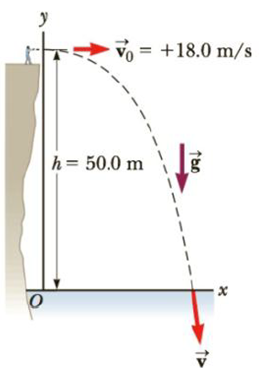Chapter 3, Problem 7P

Chapter
Section
Textbook Problem

A student stands at the edge of a cliff and throws a stone horizontally over the edge with a speed of 18.0 m/s. The cliff is 50.0 m above a flat, horizontal beach as shown in Figure P3.7. (a) What are the coordinates of the initial position of the stone? (b) What are the components of the initial velocity? (c) Write the equations for the x- and y-components of the velocity of the stone with time, (d) Write the equations for the position of the stone with time, using the coordinates in Figure P3.7. (e) How long after being released does the stone strike the beach below the cliff? (f) With what speed and angle of impact does the stone land?Figure P3.7

(a)

To determine
The coordinates of the initial position of the stone.

Explanation

Initially, as the student is standing at the top of the cliff, the coordinate of the stone is zero and the y coordinate of the stone will be equal to the height of the cliff.

Conclusion:

Thus, the coordinates of the

(b)

To determine
The components of the initial velocity of the stone.

(c)

To determine
The equations for x and y components of the velocity of the stone with time.

(d)

To determine
The equations for the position of the stone with time.

(e)

To determine
The time taken for the stone to strike the beach.

(f)

To determine
The speed and angle of impact of the stone when it lands.

Still sussing out bartleby?

Check out a sample textbook solution.

See a sample solution

The Solution to Your Study Problems

Bartleby provides explanations to thousands of textbook problems written by our experts, many with advanced degrees!

Get Started